### Transient Vibrations: Response of Spring–Mass System to a Ramp Function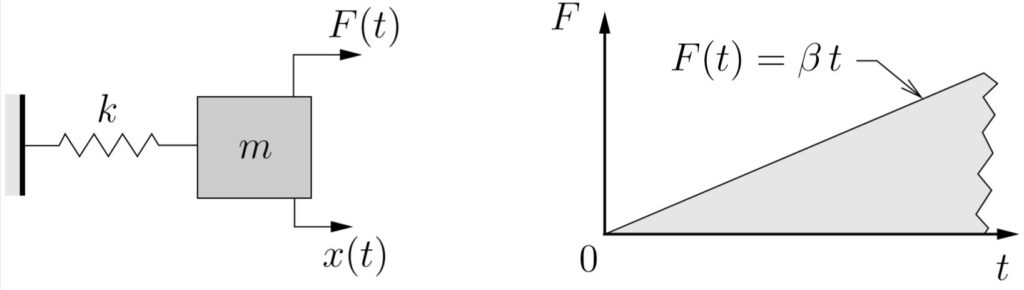Ramp Function

The equation of motion in this case is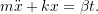It is easy to verify by direct substitution that the particular solution is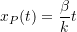so the total solution is

(7.5)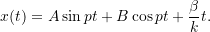If the initial conditions are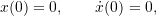then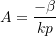and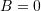so we get

(7.6)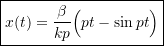which is illustrated below.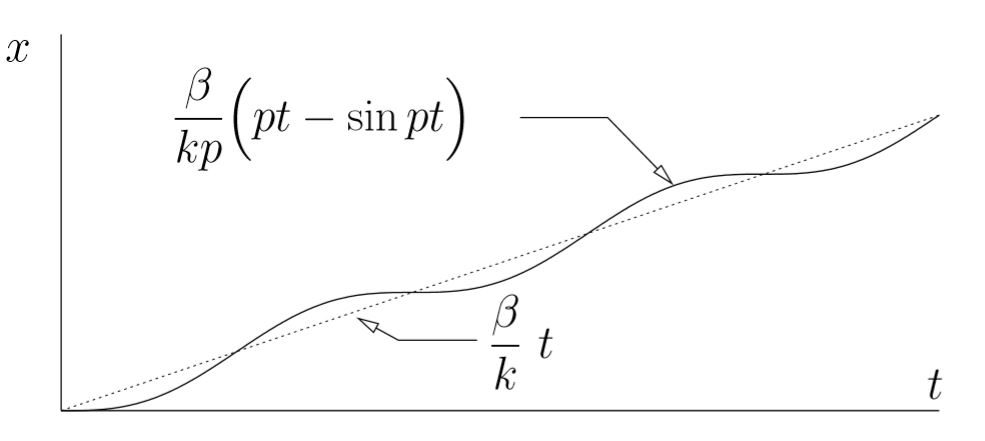Response to Ramp Function with x(0) = ˙x(0) = 0

We can interpret this response as an oscillation with amplitude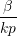about an equilibrium position that is increasing linearly with time.